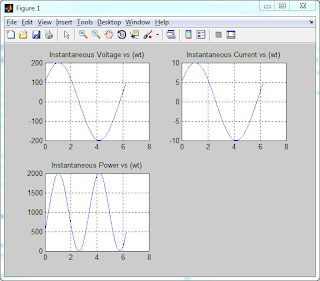Hello Friends, Today I had an exam in a Power System Course, so just got little excited about Power System and related analysis in MATLAB.

In this post, I want to share with you a very basic MATLAB function which I created recently. Given the values of Voltage and Impedance, this function can plot the graphs of Instantaneous Voltage, Instantaneous Current and Instantaneous Power.

The MATLAB code is given below. Alternatively you can download the M-file for the function from here:

Just download the zipped file, unzip it and open the 'graph.m' file. If your MATLAB console's current directory is set to the folder in which the graph.m file is present, then you can also view the syntax and explanation of this function by typing
help graph
in the command window.

The sample output graph is shown:Voltage, Current, Power vs Time Graphs

The MATLAB code is given below:

``````
%THIS FUNCTION PLOTS 3 GRAPHS
%1. INSTANTANEOUS VOLTAGE
%2. INSTANTANEOUS CURRENT
%3. INSTANTANEOUS POWER
%USER INPUT ARE ABSOLUTE VALUE OF VOLTAGE, VOLTAGE ANGLE,
% ABSOLUTE VALUE OF IMPEDANCE AND IMPEDANCE ANGLE
%-----------------------------------------------------
%Syntax:    graph(Vm,Vangle,Z,Zangle)
%Example:   graph(100,0,2,60)
%-----------------------------------------------------
%PROGRAMMED BY: AMIT BISWAL
%URL: WWW.123MYLIST.COM
%-----------------------------------------------------
function graphs=graph(Vm,Vangle,Z,Zangle)
clc
wt=0:0.01:(2*pi);
v=Vm*cos(wt-((pi/180)*Vangle));
z=Z*exp(j*(pi/180)*Zangle);
i=v/z;
xline=zeros(1,length(wt));
power=v.*i;
subplot(2,2,1);
plot(wt,v);
title('Instantaneous Voltage vs (wt)')
grid
subplot(2,2,2);
plot(wt,i);
title('Instantaneous Current vs (wt)');
grid
subplot(2,2,3);
plot(wt,power);
title('Instantaneous Power vs (wt)');
grid

``````